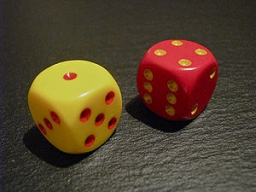# Probability 41101

What is the probability of a random event A that
a) an even number,
b) a number divisible by three,
c) a number greater than six will fall

on the dice roll.

p1 =  0.5
p2 =  0.3333
p3 =  0

### Step-by-step explanation:Did you find an error or inaccuracy? Feel free to write us. Thank you!

Tips for related online calculators
Would you like to compute the count of combinations?